## What is a geometry?

### Introduction

Vector data consists of geometries such as points, lines, polygons, circles, and so on. Examples of vector data are buildings, roads, railways, stations, and so on.

### Geometry terminology

Curve

A curve is a continuous, one-dimensional shape. You can retrieve its points using a single parameter. A curve may consist itself of other curves, for example curve segments.

Curve segment

1-dimensional geometric object for representing a continuous component of a curve using homogeneous interpolation and definition methods. It is the simplest form of a curve.

Point

0-dimensional geometric primitive, representing a position.

Ring

A ring is a closed curve, meaning that its start and end points are the same.

Surface

A surface is a continuous, 2-dimensional shape. Its exterior boundary, which separates it from infinite space, is defined by a single ring. A surface may contain zero or more holes, all of which are also defined using rings.

## Geometry model

The following sections show the hierarchy for the geometry model. The diagrams are not exhaustive.

### Basis for the geometry model

Every geometry is defined with respect to a coordinate reference.

Geometries can be categorized according to their dimensions.

• Point: 0-dimensional geometry

• Curve: 1-dimensional geometry

• Surface: 2-dimensional geometry

• Solid: 3-dimensional geometry (not included in the diagram)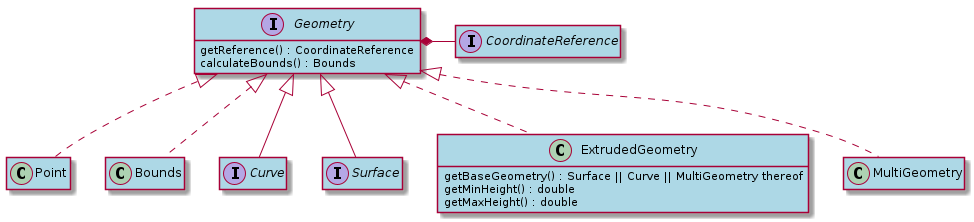#### Curves

The diagram shows the hierarchy for the curve geometries.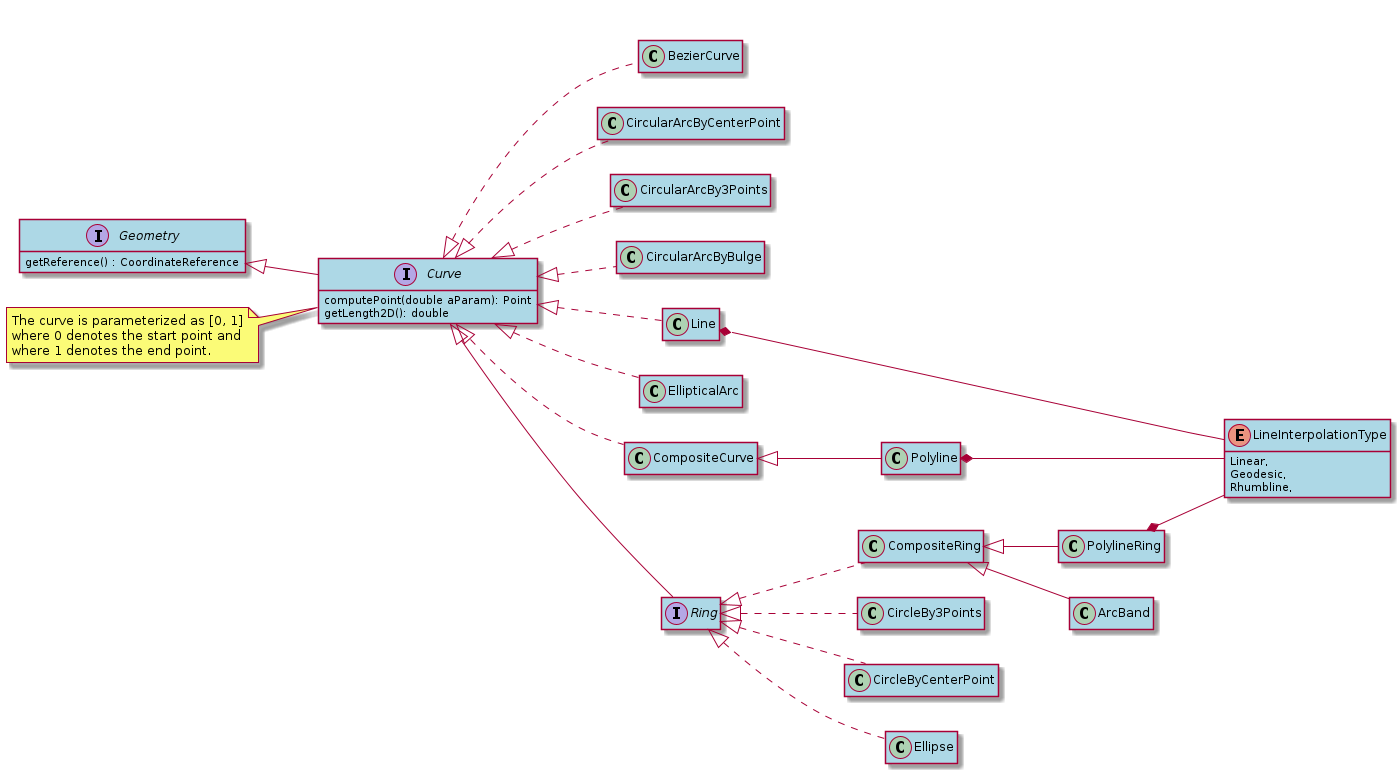#### Surfaces

Surface terminology:

Patch

A patch is a surface based on a single ring, thus not having any holes.

CompositePatch

A composite patch is a general surface that is based on an exterior patch and zero or more inner patches. The inner patches represent holes within the surface. The patch rings should not intersect.

The diagram shows the hierarchy for the surface geometries.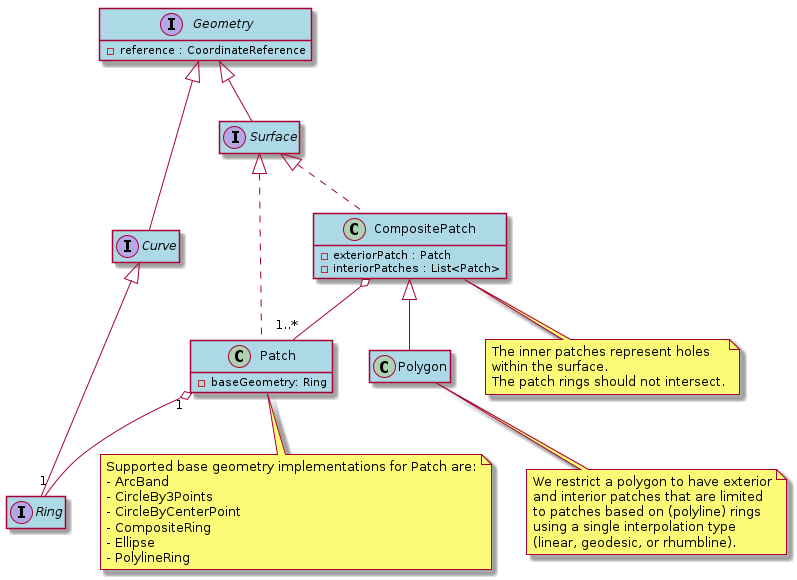#### Geometry aggregates

The diagram shows the hierarchy for aggregates of geometries.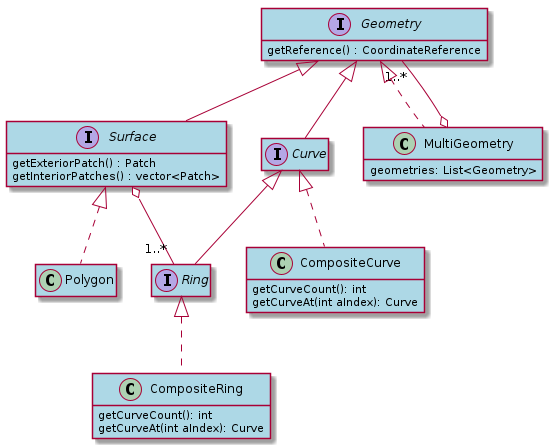## Common geometries

You have access to the following commonly used geometries. For each of the geometries, there is a link to the factory method to create the geometry.

Point

A single point location. See `GeometryFactory::createPoint`.

Line

A line defined by exactly 2 points. See `GeometryFactory::createLine`.

Polyline

A line defined by multiple points.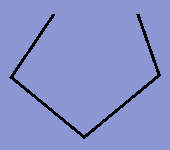Figure 1. Polyline
PolylineRing

A line defined by multiple points representing a ring; thus the start and the end point are always the same.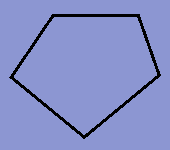Figure 2. Polyline ring
Circle

All of its points are located on a circle with a center point and a radius.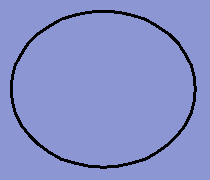Figure 3. Circle

A circle can be defined in two ways:

Circular arc

All of its points are located on a circle with a center point and a radius. The arc starts at a start angle and ends at an end angle.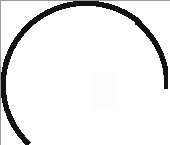Figure 4. Circular arc

A circular arc can be defined in three ways:

Ellipse

All of its points are located on an ellipse defined by a center point, semi-major axis length, semi-minor axis length and a rotation angle.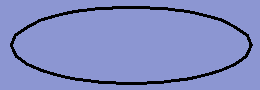Figure 5. Ellipse

An ellipse can be created using `GeometryFactory::createEllipse`.

Elliptical arc

All of its points are located on an ellipse with a center point, semi-major axis, semi-minor axis and a rotation angle. The arc starts at a start angle and ends at an end angle.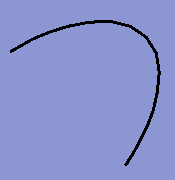Figure 6. Elliptical arc
Arc band

All of its points are located on the band with a minimum radius and a maximum radius from the focus point. The arc band starts at a start angle and ends at an end angle.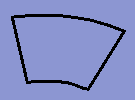Figure 7. Arc band
Bounds

A box with a location (Point), a width, a height, and a depth. Bounds is often used as a bounding box for more complex geometries.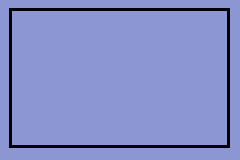Figure 8. Bounds
Patch

A patch is a surface with an exterior is based on a ring. It has no holes.

CompositePatch

A composite patch is a surface based on an exterior patch and zero or more interior patches. The patch rings do not intersect.

Polygon

A polygon is a surface which has an exterior and 0 or more interiors, which represent holes. The exterior and the interior boundaries are closed polyline geometries (polyline ring).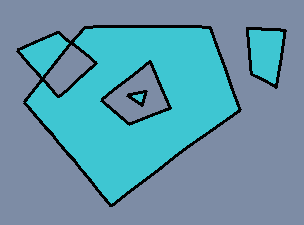Figure 9. Polygon
Extruded geometry

An extruded geometry is a 3D shape created by extruding a 2D Curve or Surface with a minimum and maximum height. Currently, all Curve or Surface instances are supported bases for an extruded geometry, as well as any MultiGeometry that consists solely of such geometries. The image below shows an extruded ellipse.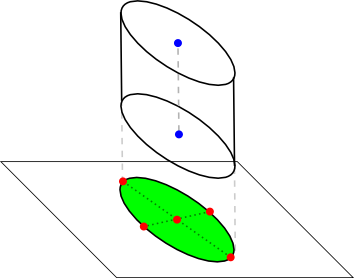Figure 10. Extruded geometry
Multi geometry

A multi-geometry is a collection of one or more geometry objects.

## Modifying geometries

Geometries are immutable by design, so instances cannot be modified directly. You can programmatically create a modified Geometry instance by:

• using GeometryFactory with modified construction parameters

• using a method on the Geometry class that adapts a part of the geometry and returns a new instance. Examples are:

Note: for interactive creation and editing of geometries (and features), see:

## Constraining geometries

The methods on the Geometry classes to make modifications and the GeometryFactory class do not enforce any constraints. It is the user’s responsibility to do so. To help you with this, constraint interfaces are available for every geometry type. See for example

You can implement these, and apply them where needed. This can be done by creating or modifying a geometry, and then applying the constraint.

Program (C++): Constraining a geometry using the API.
``````// Create a new Polyline and constrain it
auto constraint = std::make_shared<MyPolylineConstraint>();
auto polyline = GeometryFactory::createPolyline(reference, coordinates, LineInterpolationType::Geodesic);
auto constrainedPolyline = constraint->apply(polyline);

// Modify a Polyline and constrain it
auto modifiedPolyline = polyline->translate(translation);
auto polylineChange = PolylineChange();
polylineChange.setTranslation(translation);
constrainedPolyline = constraint->apply(polyline, modifiedPolyline, polylineChange);``````

C#

``````// Create a new Polyline and constrain it
var constraint = new MyPolylineConstraint();
var polyline = GeometryFactory.CreatePolyline(reference, coordinates, LineInterpolationType.Geodesic);
var constrainedPolyline = constraint.Apply(polyline);

// Modify a Polyline and constrain it
var modifiedPolyline = polyline.Translate(translation);
var polylineChange = new PolylineChange {Translation = translation};
constrainedPolyline = constraint.Apply(polyline, modifiedPolyline, polylineChange);``````

Note that interactive editing and creation have out of the box support for constraints. See the related article for more information.